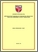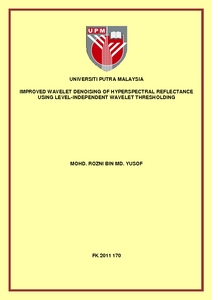# Improved wavelet denoising of hyperspectral reflectance using level-independent wavelet thresholding

## Citation

Md Yusof, Mohd Rozni (2011) Improved wavelet denoising of hyperspectral reflectance using level-independent wavelet thresholding. PhD thesis, Universiti Putra Malaysia.

## Abstract

A method for preconditioning vegetation reflectance spectra prior to applying wavelet denoising methods is presented. Various methods for denoising signals exhibiting Poisson noise characteristics using wavelet thresholding have been proposed; however,these methods focus on the actual count of photons or electrons or some number directly proportional to them. These methods may be less useful for denoising vegetation reflectance spectra, since reflectance is defined as a ratio of the reflected radiance and the incident irradiance, taking the value between 0 and 1. It is proposed here to precondition the reflectance signal by multiplying it by a constant that reflects the number of photons detected, prior to applying the Anscombe Transform and wavelet thresholding, in order to make wavelet denoising methods more suitable for reflectance spectra. This constant is to be calculated from the noise variance of the square root of the signal and is a nominal value of the signal radiance. The signal, having been denoised, is subjected to the inverse Anscombe Transform and divided by this constant to obtain the clean reflectance spectrum. The method has been tested on a simulated vegetation reflectance spectrum created using the PROSPECT leaf model and a Poisson noise model, and later on real world signals of different spectral sampling intervals, and is found to satisfactorily denoise vegetation reflectance spectra. Furthermore, the performance in terms of RMSE in the simulation reaches a plateau as excessive levels of wavelet decomposition are used. The visual evaluation of both the synthetic spectra and real world signals used also indicate that this method does not degrade the signal if excessive levels of wavelet decomposition are used for denoising. As level of decomposition is a very important parameter in wavelet denoising, which can result in oversmoothing if not determined correctly, and it is hard to know the correct optimum value a priori, this method simplifies the wavelet denoising process by making the precise number of level of decompositions to use non-critical.Preview
PDF
FK 2011 170R.pdfView Item